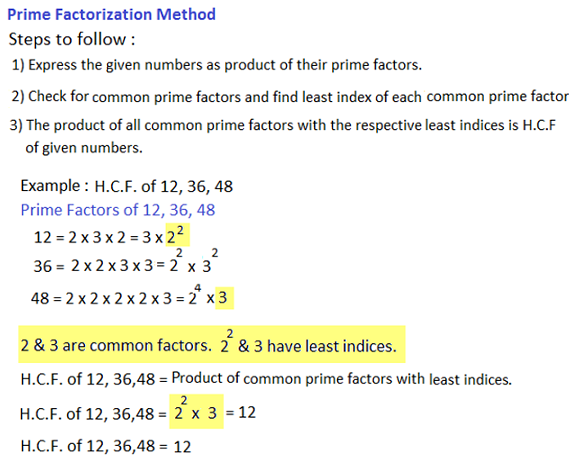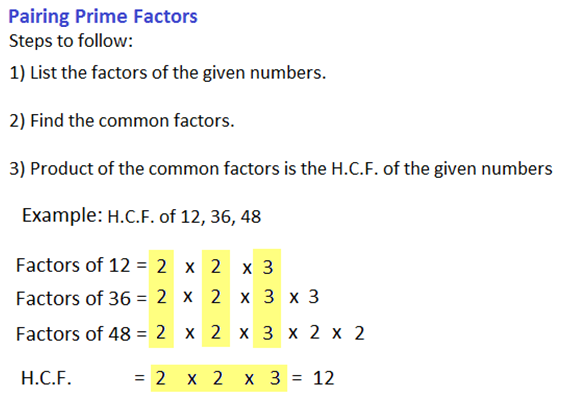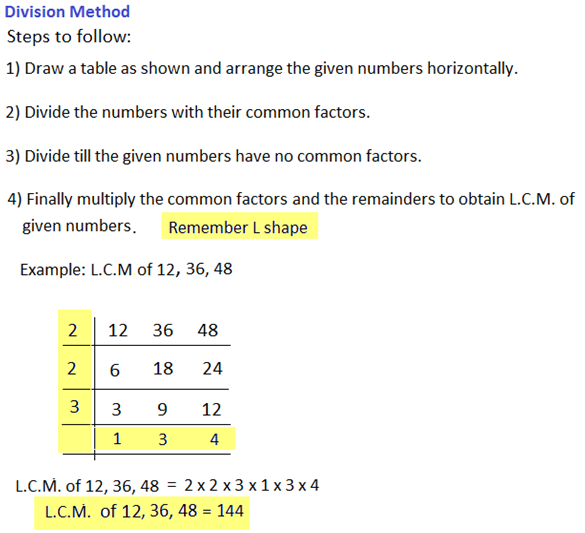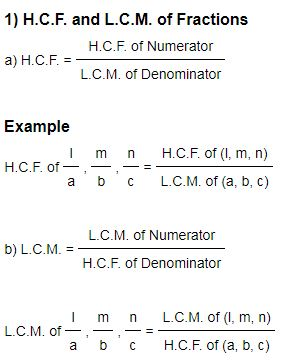# Highest Common Factor And Least Common Multiple

### Why are H.C.F and L.C.M important?

The chapter of H.C.F and L.C.M is very important. This concept is useful in the chapters of time and distance, time and work, pipes and cisterns, etc. The tricks used to find L.C.M and H.C.F of two or more numbers help in finding out quick solutions and thus reduce time during exams.

L.C.M. concept is important to solve problems related to racetracks, traffic lights, etc. H.C.F. concept is useful in calculating the largest size of tile/room in particular area, largest tape to measure the land, etc.

#### Important terms

1) Factors: Factor is a number which exactly divides other number.

Example: 3 and 5 are factors of 15

2) Multiple: A number is said to be multiple of another number when it is exactly divisible by another number.

Example: 15 is a multiple of 3 and 5

3) Common multiple: A common multiple of two or more numbers is a number which is exactly divisible by each of them.

Example: 18 is a common multiple of 2,3,6 and 9

4) H.C.F/G.C.F:M/b> (Highest Common Factor / Greatest Common Factor). H.C.F of two or more numbers is the greatest number which divides each number exactly.

5) L.C.M.: (Lowest common multiple). The least number exactly divisible by each one of the given numbers is called least common multiple.

### Points To Remember

Before studying this chapter, make sure that the basic concepts of numbers (prime numbers, composite numbers, co-prime numbers, etc) are perfectly understood. Knowing basics will help in understanding this chapter.

#### Quick Revision of prime numbers, composite numbers and co-prime numbers is given below### H.C.F

• It is the greatest number which exactly divides all the given numbers.
• The H.C.F of two or more numbers is smaller than or equal to the smallest number of given numbers.
• H.C.F of given numbers divides their L.C.M.
• Two numbers are said to be co-prime if their H.C.F. is 1.

### L.C.M

• To find L.C.M. of two co-prime numbers, just multiply the given two numbers.
• The L.C.M. of two or more numbers is greater than or equal to the greatest number of given numbers.

## Methods to Find H.C.F. of Given Numbers### Tips and Tricks#### 2) Product of two numbers = Product of their H.C.F. and L.C.M.

This condition is only true for two given numbers. If H.C.F. and L.C.M. of three or more numbers are given, then this rule is not applicable.

#### H.C.F. and L.C.M. of decimal fractions

To find H.C.F. or L.C.M. of 0.5, 1.6, etc, consider these numbers without decimal point i.e 5, 16 and solve using normal methods used to determine H.C.F. or L.C.M.. In the result, mark off as many decimal places as there are in each of the given number.

#### Comparison of fractions

Find L.C.M. of denominators of given fractions. Convert each fraction into equivalent fraction with L.C.M. as denominator, by multiplying both numerator and denominator by same number. The resultant fraction with the greatest numerator is greatest.

### About us

EntryTest.com is a free service for students seeking successful career.CAT - College of Admission Tests. All rights reserved. College of Admission Tests Online Test Preparation The CAT Online